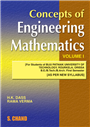Home > Tech Professional > Applied Science > Introduction to Engineering Mathematics - Volume IV# Introduction to Engineering Mathematics - Volume IV

## H K Dass & Dr. Rama Verma

 ISBN : 9789352837465 Pages : 448 Binding : Paperback Language : English Imprint : S. Chand Publishing Trim size : 6.75 X 9.5 inches © year : 2019
 List Price :325.00
 Offer Price :260.00

20% OFF

# In Stock

Credit Card / Debit Card / Internet BankingIntroduction to Engineering Mathematics - Volume IV has been thoroughly revised according to the New Syllabi (2018 onwards) of Dr. A.P.J. Abdul Kalam Technical University (AKTU, Lucknow). The book contains 13 chapters divided among five modules - Partial Differential Equations, Applications of Partial Differential Equations, Statistical Techniques - I, Statistical Techniques - II and Statistical Techniques - III.  With close to 400 Examples and 700 questions the textbook takes care of the understanding of concepts as well as retention and practice. Since it is the first-time examinations would be held for this course, there are two book-end Model Test Papers so that the students may get the practice and not find any difficulty while answering their final examinations. Module - I: Partial Differential Equations 1. Linear and Non-Linear Partial Differential Equations 2. Linear Partial Differential Equations of Higher Order with Constant Coefficients 3. Equations Reducible to Linear Partial Differential Equations with Constant Coefficients Module - II: Applications of Partial Differential Equations 4. Partial Differential Equations: Applications Module - III: Statistical Techniques - I 5. Statistical Techniques 6. Curve Fitting (Method of Least Squares) 7. Correlation and Regression Module - IV: Statistical Techniques - II 8. Probability and Distribution 9. Binomial Distribution 10. Poisson Distribution 11. Normal Distribution Module - V: Statistical Techniques - III 12. Tests of Significance (t-Test, F-Test, Chi Square Test) 13. Analysis of Variance and Statistical Quality Control Model Test Paper - I Model Test Paper - II This book is designed for undergraduate students of engineering courses of Dr. A.P.J. Abdul Kalam Technical University (AKTU, Lucknow).### More Books By Author#### S Chand's New Mathematics for ...

9789352832569530.00424.00

20% OFF#### Concepts of Engineering ...

9788121923576125.00100.00

20% OFF325.00260.00